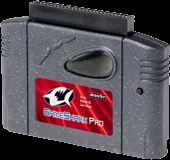How Gameshark codes workMost Gameshark users simply enter the codes and use them.  And there's nothing wrong with that.   But for every 10 people like that, there's one person who goes, "now how does it do that!?"  This section is for those people.

Hexidecimal-  This is very simple.  You notice that when you are entering codes, there are numbers and letters.  So hexidecimal is the number system that the Gameshark uses.
Decimal, the system we normally use, goes like this:
0 1 2 3 4 5 6 7 8 9 10 11 12 13 14 15 etc.
But Hexidecimal is different, it goes like tis:
0 1 2 3 4 5 6 7 8 9 A B C D E F 10 11 etc.
So the highest two digit number in Hexidecimal is FF, which is 255 in Dedcimal.  You will see this value frequently.

What codes actually are-  All of the information in the game is stored in order.  And each spot in the memory is called an address.  The beginings of most Gameshark codes are the addresses.  The end of the address is what value that address has.  So, lets say you have a code that changes how many bullets you have.  Lets say that code is 80056349 0012.  The address in the memory that has the amount of bullets is 80056349.  And the value there is 12, and that 12 is in Hexidecimal, so it's really a 18 in Decimal.

Types of codes-  The type that a code is is determined by the begining.  Most codes start with 80.  That is a regular 8-bit code.  If a code starts with 81, than it is a 16-bit code.  That means that it is really 2 8-bit codes in one.  Lets say you have a 16-bit code, 81072940 4398.  That 16-bit codes is both 80072940 0043 and 80072940 in one.  So the codes have to be in order to make it a 16-bit code.  And it has to be even.  But these codes are still very useful in long codes.

Another type of code is an activator.  These types of codes start with D0.  When you enter a code that has an activator in it, an d there is a line of code below that activator, the activator effects that line, and that line only.  The activator is activated whenyou press the correct button on your Gameshark.  That button is determined by the end of the code.  Each digit means that the activator works with a different button.  Activator codes are different for each game.  So here's an example.  Let's use that ammo code from before.  So you have this entered in your Gameshark:
D0064824 0050
80056349 0012
So if the 50 makes the activator work with the C-left button, when you press the C-left button the code will be activated, and you will get the 18 bullets.

Making codes from the memory editor-  "I've made some changes in the memory editor, but how do I make it into codes?"  That is a question that I get a lot.  So I have answered it right here, using this picture.  It's from the Terrorist's Goldeneye Site.Now. Making codes from this.  Let's say you just changed that "happy now karl?" text.  You see the address on the left.  The first pair of numbers on the left are for that address.  So the first code on the third to last line would be 802AF180 000A.  The next one would be 802AF181 0000.  So the  addresses for "Happy now karl?" would start at 802AF184, and would go on.  If you wanted to make a code that affected the H and the A in "happy," you could use a 16-bit code.  It would be 812AF184 6861.  Got it?